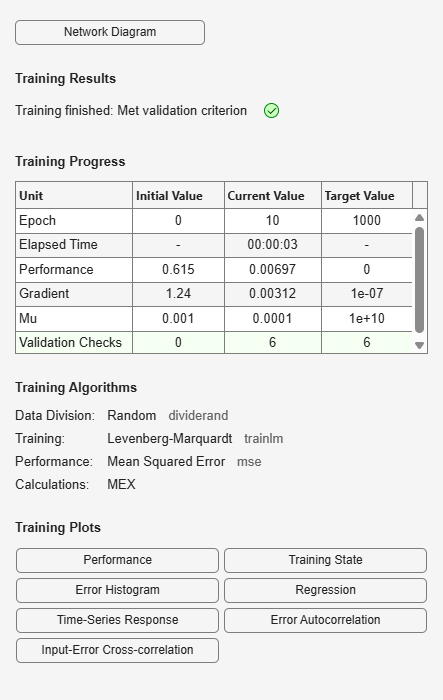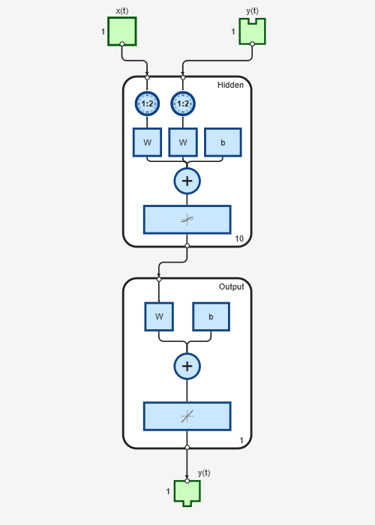# narxnet

Nonlinear autoregressive neural network with external input

## Syntax

``narxnet(inputDelays,feedbackDelays,hiddenSizes,feedbackMode,trainFcn)``

## Description

example

````narxnet(inputDelays,feedbackDelays,hiddenSizes,feedbackMode,trainFcn)` takes these arguments: Row vector of increasing 0 or positive input delays, `inputDelays`Row vector of increasing 0 or positive feedback delays, `feedbackDelays`Row vector of one or more hidden layer sizes, `hiddenSizes`Type of feedback, `feedbackMode`Backpropagation training function, `trainFcn` and returns a NARX neural network. NARX (Nonlinear autoregressive with external input) networks can learn to predict one time series given past values of the same time series, the feedback input, and another time series called the external (or exogenous) time series.```

## Examples

collapse all

Train a nonlinear autoregressive with external input (NARX) neural network and predict on new time series data. Predicting a sequence of values in a time series is also known as multistep prediction. Closed-loop networks can perform multistep predictions. When external feedback is missing, closed-loop networks can continue to predict by using internal feedback. In NARX prediction, the future values of a time series are predicted from past values of that series, the feedback input, and an external time series.

Load the simple time series prediction data.

`[X,T] = simpleseries_dataset;`

Partition the data into training data `XTrain` and `TTrain`, and data for prediction `XPredict`. Use `XPredict` to perform prediction after you create the closed-loop network.

```XTrain = X(1:80); TTrain = T(1:80); XPredict = X(81:100);```

Create a NARX network. Define the input delays, feedback delays, and size of the hidden layers.

`net = narxnet(1:2,1:2,10);`

Prepare the time series data using `preparets`. This function automatically shifts input and target time series by the number of steps needed to fill the initial input and layer delay states.

`[Xs,Xi,Ai,Ts] = preparets(net,XTrain,{},TTrain);`

A recommended practice is to fully create the network in an open loop, and then transform the network to a closed loop for multistep-ahead prediction. Then, the closed-loop network can predict as many future values as you want. If you simulate the neural network in closed-loop mode only, the network can perform as many predictions as the number of time steps in the input series.

Train the NARX network. The `train` function trains the network in an open loop (series-parallel architecture), including the validation and testing steps.

`net = train(net,Xs,Ts,Xi,Ai);`Display the trained network.

`view(net)`Calculate the network output `Y`, final input states `Xf`, and final layer states `Af` of the open-loop network from the network input `Xs`, initial input states `Xi`, and initial layer states `Ai`.

`[Y,Xf,Af] = net(Xs,Xi,Ai);`

Calculate the network performance.

`perf = perform(net,Ts,Y)`
```perf = 0.0153 ```

To predict the output for the next 20 time steps, first simulate the network in closed-loop mode. The final input states `Xf` and layer states `Af` of the open-loop network net become the initial input states `Xic` and layer states `Aic` of the closed-loop network `netc`.

`[netc,Xic,Aic] = closeloop(net,Xf,Af);`

Display the closed-loop network.

`view(netc)`Run the prediction for 20 time steps ahead in closed-loop mode.

`Yc = netc(XPredict,Xic,Aic)`
```Yc=1×20 cell array {[-0.0156]} {[0.1133]} {[-0.1472]} {[-0.0706]} {[0.0355]} {[-0.2829]} {[0.2047]} {[-0.3809]} {[-0.2836]} {[0.1886]} {[-0.1813]} {[0.1373]} {[0.2189]} {[0.3122]} {[0.2346]} {[-0.0156]} {[0.0724]} {[0.3395]} {[0.1940]} {[0.0757]} ```

## Input Arguments

collapse all

Zero or positive input delays, specified as an increasing row vector.

Zero or positive feedback delays, specified as an increasing row vector.

Sizes of the hidden layers, specified as a row vector of one or more elements.

Type of feedback, specified as either `'open'`, `'closed'`, or `'none'`.

Training function name, specified as one of the following.

Training FunctionAlgorithm
`'trainlm'`

Levenberg-Marquardt

`'trainbr'`

Bayesian Regularization

`'trainbfg'`

BFGS Quasi-Newton

`'trainrp'`

Resilient Backpropagation

`'trainscg'`

`'traincgb'`

`'traincgf'`

`'traincgp'`

`'trainoss'`

One Step Secant

`'traingdx'`

`'traingdm'`

`'traingd'`

Example: For example, you can specify the variable learning rate gradient descent algorithm as the training algorithm as follows: `'traingdx'`

For more information on the training functions, see Train and Apply Multilayer Shallow Neural Networks and Choose a Multilayer Neural Network Training Function.

Data Types: `char`

## Version History

Introduced in R2010b